# 如何判断一个多边形是否合法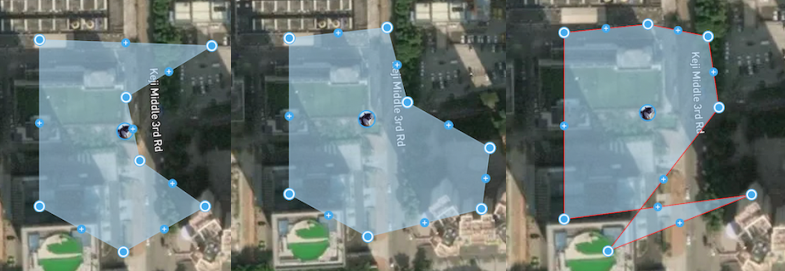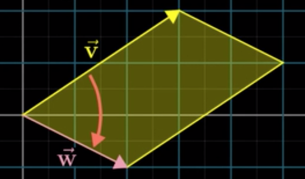$$\vec{v} \times \vec{w} < 0$$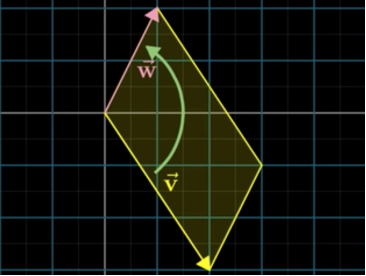$$\vec{v} \times \vec{w} > 0$$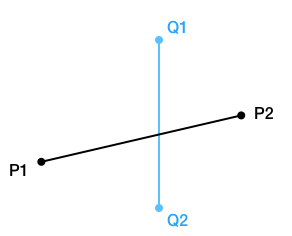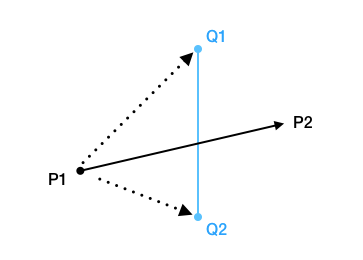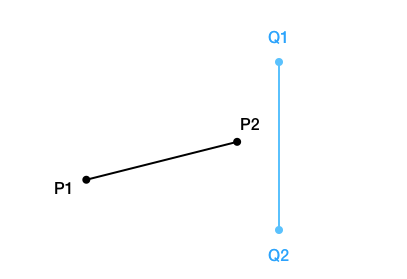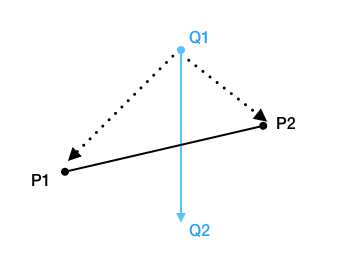A = $\vec{P_1P_2} \times \vec{P_1Q_1}$
B = $\vec{P_1P_2} \times \vec{P_1Q_2}$
C = $\vec{Q_1Q_2} \times \vec{Q_1P_1}$
D = $\vec{Q_1Q_2} \times \vec{Q_1P_2}$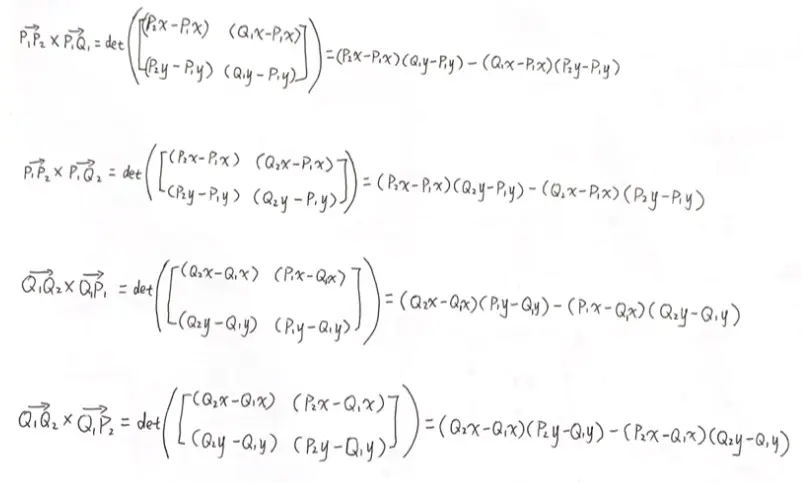HansonTalk iOSTypist